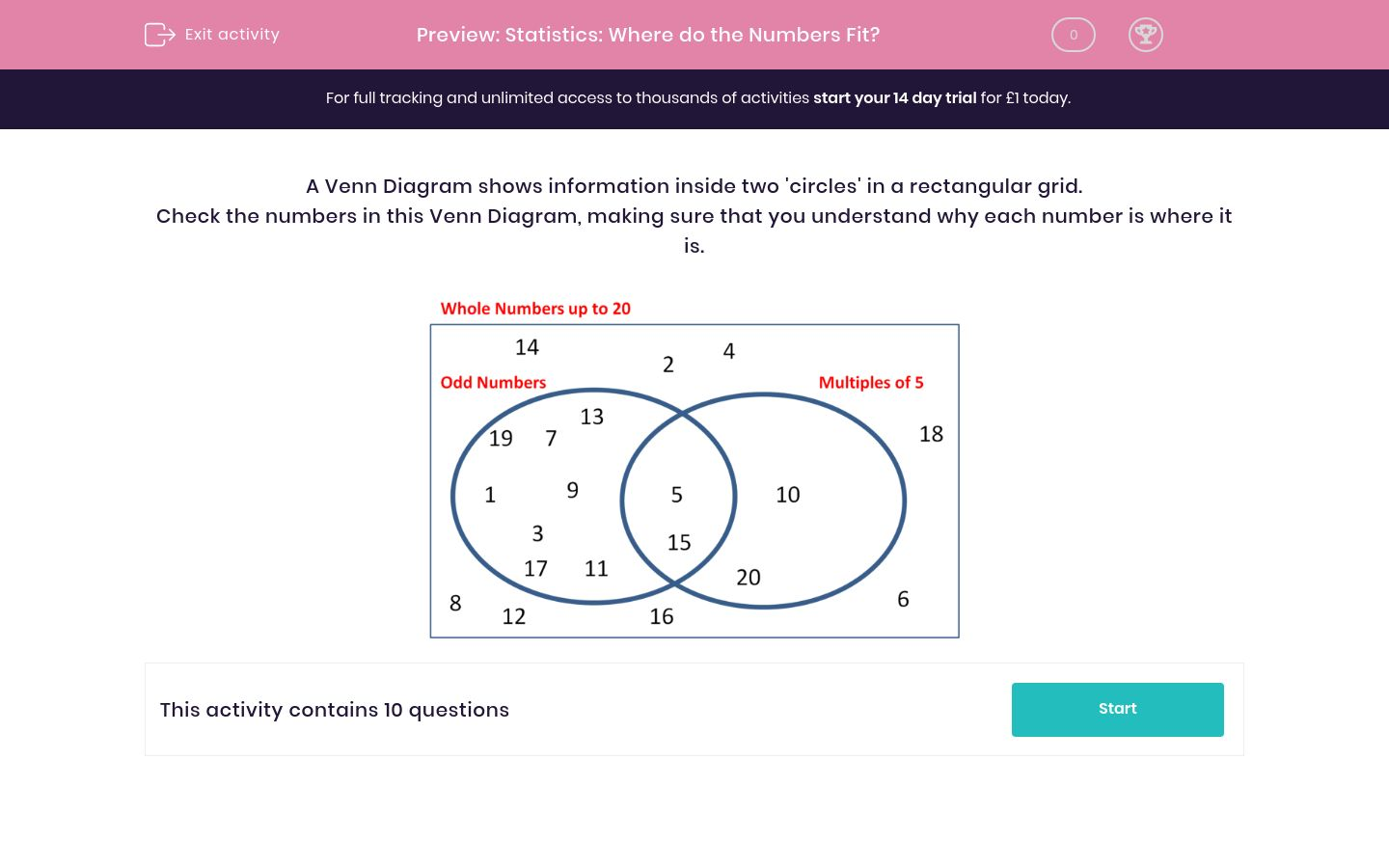# Statistics: Where do the Numbers Fit?

In this worksheet, students answer questions related to a diagram.Key stage:  KS 2

Curriculum topic:   Maths and Numerical Reasoning

Curriculum subtopic:   Interpreting Data

Difficulty level:### QUESTION 1 of 10

A Venn Diagram shows information inside two 'circles' in a rectangular grid.

Check the numbers in this Venn Diagram, making sure that you understand why each number is where it is.In which section will the number 10 go in this Venn Diagram?A

B

C

D

In which section will the number 20 go in this Venn Diagram?A

B

C

D

In which section will the number 30 go in this Venn Diagram?A

B

C

D

In which section will the number 26 go in this Venn Diagram?A

B

C

D

In which section will the number 27 go in this Venn Diagram?A

B

C

D

In which section will the number 27 go in this Venn Diagram?A

B

C

D

In which section will the number 30 go in this Venn Diagram?A

B

C

D

In which section will the number 3 go in this Venn Diagram?A

B

C

D

In which section will the number 21 go in this Venn Diagram?A

B

C

D

Which number will go into section B?• Question 1

In which section will the number 10 go in this Venn Diagram?C
• Question 2

In which section will the number 20 go in this Venn Diagram?C
• Question 3

In which section will the number 30 go in this Venn Diagram?B
• Question 4

In which section will the number 26 go in this Venn Diagram?D
• Question 5

In which section will the number 27 go in this Venn Diagram?A
• Question 6

In which section will the number 27 go in this Venn Diagram?D
• Question 7

In which section will the number 30 go in this Venn Diagram?A
• Question 8

In which section will the number 3 go in this Venn Diagram?D
• Question 9

In which section will the number 21 go in this Venn Diagram?C
• Question 10

Which number will go into section B?